Measures of Variation

Range

The range is the simplest measure of variation to find. It is simply the highest value minus the lowest value.

RANGE = MAXIMUM - MINIMUM

Since the range only uses the largest and smallest values, it is greatly affected by extreme values, that is - it is not resistant to change.

Variance

"Average Deviation"

The range only involves the smallest and largest numbers, and it would be desirable to have a statistic which involved all of the data values.

The first attempt one might make at this is something they might call the average deviation from the mean and define it as: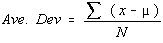The problem is that this summation is always zero. So, the average deviation will always be zero. That is why the average deviation is never used.

Variation

So, to keep it from being zero, the deviation from the mean is squared and called the "squared deviation from the mean". The sum of the squared deviations from the mean is called the variation. The problem with the variation is that it does not take into account how many data values were used to obtain the sum.

Population Variance

If we divide the variation by the number of values in the population, we get something called the population variance. This variance is the "average squared deviation from the mean".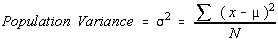Unbiased Estimate of the Population Variance

One would expect the sample variance to simply be the population variance with the population mean replaced by the sample mean. However, one of the major uses of statistics is to estimate the corresponding parameter. This formula has the problem that the estimated value isn't the same as the parameter. To counteract this, the sum of the squares of the deviations is divided by one less than the sample size.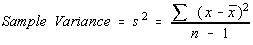Standard Deviation

There is a problem with variances. Recall that the deviations were squared. That means that the units were also squared. To get the units back the same as the original data values, the square root must be taken.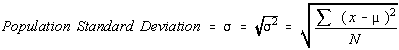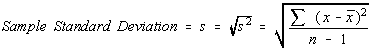The sample standard deviation is not the unbiased estimator for the population standard deviation.

The calculator does not have a variance key on it. It does have a standard deviation key. You will have to square the standard deviation to find the variance.

Sum of Squares (shortcuts)

The sum of the squares of the deviations from the means is given a shortcut notation and several alternative formulas.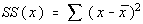A little algebraic simplification returns: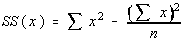What's wrong with the first formula, you ask? Consider the following example - the last row are the totals for the columns

1. Total the data values: 23
2. Divide by the number of values to get the mean: 23/5 = 4.6
3. Subtract the mean from each value to get the numbers in the second column.
4. Square each number in the second column to get the values in the third column.
5. Total the numbers in the third column: 5.2
6. Divide this total by one less than the sample size to get the variance: 5.2 / 4 = 1.3
 x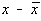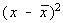4 4 - 4.6 = -0.6 ( - 0.6 )^2 = 0.36 5 5 - 4.6 = 0.4 ( 0.4 ) ^2 = 0.16 3 3 - 4.6 = -1.6 ( - 1.6 )^2 = 2.56 6 6 - 4.6 = 1.4 ( 1.4 )^2 = 1.96 5 5 - 4.6 = 0.4 ( 0.4 )^2 = 0.16 23 0.00 (Always) 5.2

Not too bad, you think. But this can get pretty bad if the sample mean doesn't happen to be an "nice" rational number. Think about having a mean of 19/7 = 2.714285714285... Those subtractions get nasty, and when you square them, they're really bad. Another problem with the first formula is that it requires you to know the mean ahead of time. For a calculator, this would mean that you have to save all of the numbers that were entered. The TI-82 does this, but most scientific calculators don't.

Now, let's consider the shortcut formula. The only things that you need to find are the sum of the values and the sum of the values squared. There is no subtraction and no decimals or fractions until the end. The last row contains the sums of the columns, just like before.

1. Record each number in the first column and the square of each number in the second column.
2. Total the first column: 23
3. Total the second column: 111
4. Compute the sum of squares: 111 - 23*23/5 = 111 - 105.8 = 5.2
5. Divide the sum of squares by one less than the sample size to get the variance = 5.2 / 4 = 1.3
 x x^2 4 16 5 25 3 9 6 36 5 25 23 111

Chebyshev's Theorem

The proportion of the values that fall within k standard deviations of the mean will be at least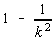, where k is an number greater than 1.

"Within k standard deviations" interprets as the interval: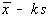to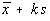.

Chebyshev's Theorem is true for any sample set, not matter what the distribution.

Empirical Rule

The empirical rule is only valid for bell-shaped (normal) distributions. The following statements are true.

• Approximately 68% of the data values fall within one standard deviation of the mean.
• Approximately 95% of the data values fall within two standard deviations of the mean.
• Approximately 99.7% of the data values fall within three standard deviations of the mean.

The empirical rule will be revisited later in the chapter on normal probabilities.

Using the TI-82 to find these values

You may use the TI-82 to find the measures of central tendency and the measures of variation using the list handling capabilities of the calculator.